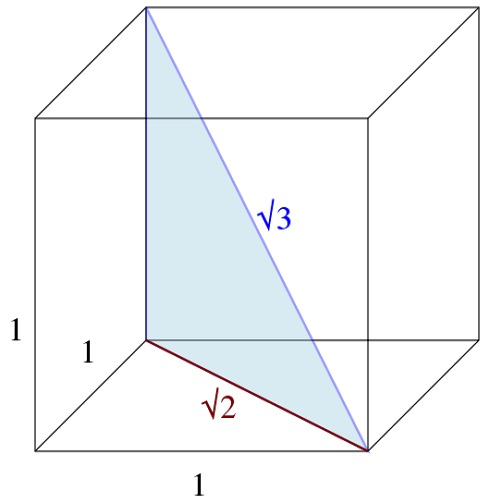Copy this diagram.To draw a cube first draw a square, then draw another square the same size that looks as if it is behind the first square, then join the vertices with four parallel lines.

Why do the diagonals of a unit cube have lengths √2 and √3?

What are the lengths of the diagonals of a cuboid with edges of length 1, 1 and 2?

What about a cuboid with edges of length 1, 2 and 3?

Use an open top cuboid shaped box. Calculate the lengths of its diagonals. Check your answers by cutting out three triangles to fit the faces of the box and a triangle to stand vertically with its hypotenuse joining opposite vertices of the cuboid like the blue triangle in the picture.

What are the lengths of the diagonals of a cuboid with edges a, b and c?